• 概率密度分布函数是不同事件发生的概率，自变量是样本取值，这样说可能不便于理解，下边通过二项分布概率公式说明： 上边是二项分布计算概率的一般公式，似然函数中的自变量是公式中的p，而概率密度分布函数中的自...

似然函数是某一特定事件发生的概率，其中自变量是分布参数θ，特定事件（一组样本取到一组特定值的联合概率）发生的概率随θ的不同而不同
概率密度分布函数是不同事件发生的概率，自变量是样本取值，这样说可能不便于理解，下边通过二项分布概率公式说明：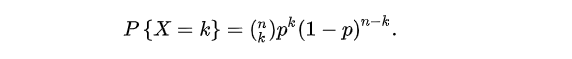上边是二项分布计算概率的一般公式，似然函数中的自变量是公式中的p，而概率密度分布函数中的自变量是公式中的k

如果你还是不理解，这里引用quora上的一个回答 What is the difference between probability and likelihood

我们可以再做一个类比，假设一个函数为 a^b ，这个函数包含两个变量。 如果你令b=2，这样你就得到了一个关于a的二次函数，即 ：a^2；当你令a=2时，你将得到一个关于b的指数函数，即 2^b
可以看到这两个函数有着不同的名字，却源于同一个函数。而p(x|θ)也是一个有着两个变量的函数。如果，你将θ设为常量，则你会得到一个概率函数（关于x的函数）；如果，你将x设为常量你将得到似然函数（关于θ的函数）

展开全文概率密度分布 似然函数 极大似然估计 概率密度函数
• 标准正态分布概率密度函数： 累积分布函数： 图像：

标准正态分布概率密度函数：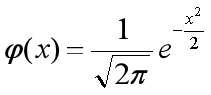累积分布函数：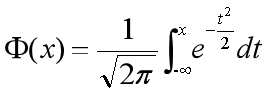图像：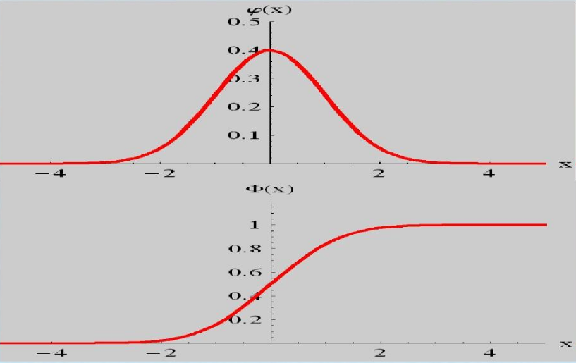展开全文• 绘制正态分布分布函数概率密度曲线 1.正态分布的概率密度函数和分布函数： 2.代码实现： import sympy import numpy as np import matplotlib.pyplot as plt # -----------构造数据-------------- # u=0 σ...

绘制正态分布的分布函数和概率密度曲线

1.正态分布的概率密度函数和分布函数：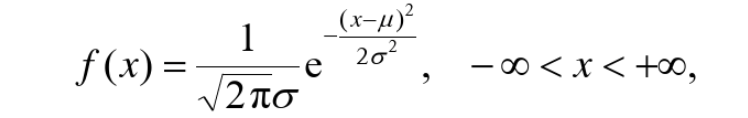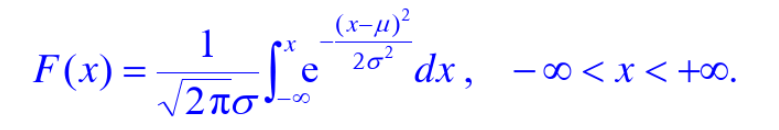2.代码实现：

import sympy
import numpy as np
import matplotlib.pyplot as plt

# -----------构造数据--------------
# u=0 σ=1
x_1, m_1 = [], []
y_1, n_1 = [], []
for i in np.arange(-10, 10, 0.1):
x_1.append(i)
y_1.append(np.exp(-(i * i / 2.0))/np.sqrt(2*np.pi))

x = sympy.symbols('x')
y = sympy.exp(-(x * x / 2.0))/sympy.sqrt(2*np.pi)
Y = sympy.integrate(y)
res = Y.subs(x, i) - Y.subs(x, -float('inf'))
m_1.append(i)
n_1.append(res)
# u=0 σ=2
x_2, m_2 = [], []
y_2, n_2 = [], []
for i in np.arange(-10, 10, 0.1):
x_2.append(i)
y_2.append(np.exp(-(i * i / (2.0*2*2)))/(np.sqrt(2*np.pi)*2))

x = sympy.symbols('x')
y = sympy.exp(-(x * x / (2.0*2*2))) / (sympy.sqrt(2 * np.pi)*2)
Y = sympy.integrate(y)
res = Y.subs(x, i) - Y.subs(x, -float('inf'))
m_2.append(i)
n_2.append(res)
# u=1 σ=1
x_3, m_3 = [], []
y_3, n_3 = [], []
for i in np.arange(-10, 10, 0.1):
x_3.append(i)
y_3.append(np.exp(-((i-1) ** 2 / 2.0)) / np.sqrt(2 * np.pi))

x = sympy.symbols('x')
y = sympy.exp(-((x-1) ** 2 / 2.0)) / sympy.sqrt(2 * np.pi)
Y = sympy.integrate(y)
res = Y.subs(x, i) - Y.subs(x, -float('inf'))
m_3.append(i)
n_3.append(res)

# --------------可视化概率密度函数---------------------------
plt.title("正态分布概率密度")                       # 设置标题
plt.rcParams['font.sans-serif'] = 'KaiTi'        # 设置字体
plt.rcParams['axes.unicode_minus'] = False       # 正常显示负号
plt.plot(x_1, y_1, c='red', label='μ=0 σ=1')     # 添加数据
plt.plot(x_2, y_2, c='green', label='μ=0 σ=2')   # 添加数据
plt.plot(x_3, y_3, c='blue', label='μ=1 σ=1')    # 添加数据
plt.xlabel('x', loc='right')                     # x轴标签
plt.ylabel('y', loc='top')                       # y轴标签
plt.legend(framealpha=1, frameon=True)           # 添加图标
plt.show()                                       # 展示

# --------------可视化分布函数---------------------------------
plt.title("正态分布分布函数")
plt.rcParams['font.sans-serif'] = 'KaiTi'
plt.rcParams['axes.unicode_minus'] = False
plt.plot(m_1, n_1, c='red', label='μ=0 σ=1')
plt.plot(m_2, n_2, c='green', label='μ=0 σ=2')
plt.plot(m_3, n_3, c='blue', label='μ=1 σ=1')
plt.xlabel('x', loc='right')
plt.ylabel('y', loc='top')
plt.legend(framealpha=1, frameon=True)
plt.show()

3.结果展示：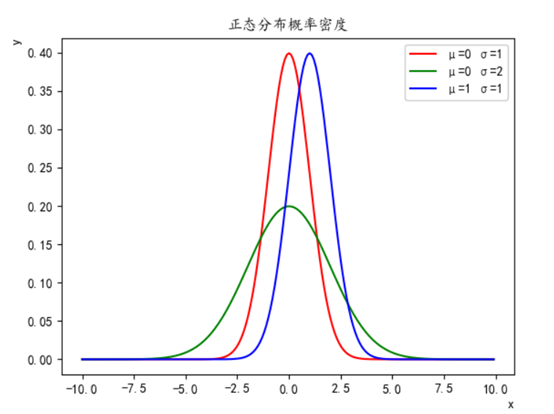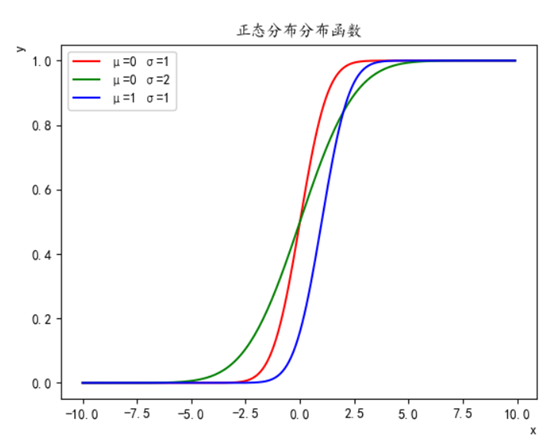展开全文数据可视化 python
• 1 先从离散型随机变量连续性随机变量说起 对于如何分辨离散型随机变量连续性随机变量，在贾俊平老师的《统计学》教材中，给出了这样的区分： 如果随机变量的值都可以逐个列举出来，则为离散型随机变量。如果随机...

1 先从离散型随机变量和连续性随机变量说起

对于如何分辨离散型随机变量和连续性随机变量，在贾俊平老师的《统计学》教材中，给出了这样的区分：

如果随机变量的值都可以逐个列举出来，则为离散型随机变量。如果随机变量X的取值无法逐个列举则为连续型变量。

进一步解释，离散型随机变量是指其数值只能用自然数或整数单位计算的则为离散变量。例如，企业个数，职工人数，设备台数等，只能按计量单位数计数，这种变量的数值一般用计数方法取得。反之，在一定区间内可以任意取值的变量叫连续变量，其数值是连续不断的，相邻两个数值可作无限分割，即可取无限个数值。例如，生产零件的规格尺寸，人体测量的身高，体重，胸围等为连续变量，其数值只能用测量或计量的方法取得。

形象点来解释：：

画一幅画，左边是梯子，右边是斜坡。
像梯子一样能说出有多少层的，可描述的，是离散型随机变量；
像斜坡一样不能说出有多少层阶梯，不可描述的，是连续性随机变量。
需要注意的是，实际操作中梯子的阶高可能很小，看起来很像斜坡，需要放大看。

2 离散型随机变量的概率函数，概率分布和分布函数

在理解概率分布函数和概率密度函数之前，我们先来看看概率函数和概率分布是咋回事。

为什么我们花这么大的力气去研究这个概念。因为它实在太重要了，为什么呢？在这里，直接引用陈希孺老师在他所著的《概率论与数理统计》这本书中说的：

研究一个随机变量，不只是要看它能取哪些值，更重要的是它取各种值的概率如何！

这句是本文的核心内容，本文的所有概念，包括概率密度，概率分布，概率函数，都是在描述概率！

2.1 概率函数和概率分布

2.1.1 概率函数

概率函数，就是用函数的形式来表达概率。

pi=P(X=ai)(i=1,2,3,4,5,6)

在这个函数里，自变量（X）是随机变量的取值，因变量（pi）是取值的概率。它就代表了每个取值的概率，所以顺理成章的它就叫做了X的概率函数。从公式上来看，概率函数一次只能表示一个取值的概率。比如P（X=1）=1/6,这代表用概率函数的形式来表示，当随机变量取值为1的概率为1/6，一次只能代表一个随机变量的取值。

2.1.1 概率分布

接下来讲概率分布，顾名思义就是概率的分布，这个概率分布还是讲概率的。我认为在理解这个概念时，关键不在于“概率”两个字，而在于“分布”这两个字。为了理解“分布”这个词，我们来看一张图。

离散型随机变量的值和概率的分布列表

在很多教材中，这样的列表都被叫做离散型随机变量的“概率分布”。其实严格来说，它应该叫“离散型随机变量的值分布和值的概率分布列表”，这个名字虽然比“概率分布”长了点，但是肯定好理解了很多。因为这个列表，上面是值，下面是这个取值相应取到的概率，而且这个列表把所有可能出现的情况全部都列出来了！

举个例子吧，一颗6面的骰子，有1，2，3，4，5，6这6个取值，每个取值取到的概率都为1/6。那么你说这个列表是不是这个骰子取值的”概率分布“？

长得挺像的，上面是取值，下面是概率，这应该就是骰子取值的“概率分布”了吧！大错特错！少了一个最重要的条件！对于一颗骰子的取值来说，它列出的不是全部的取值，把6漏掉了!

2.2 分布函数

说完概率分布，就该说说分布函数了。这个分布函数是个简化版的东西！全名应该叫概率分布函数。

看看下图中的分布律，这里的分布律明明就是我们刚刚讲的“概率函数”，完全就是一个东西。但是我知道很多教材就是叫分布律的。

概率分布函数就是把概率函数累加

我们来看看图上的公式，其中的F(x)就代表概率分布函数啦。这个符号的右边是一个长的很像概率函数的公式，但是其中的等号变成了小于等于号的公式。你再往右看看，这是一个一个的概率函数的累加！

发现概率分布函数的秘密了吗？它其实根本不是个新事物，它就是概率函数取值的累加结果！所以它又叫累积概率函数！

概率函数和概率分布函数就像是一个硬币的两面，它们都只是描述概率的不同手段！

3 连续型随机变量的概率函数和分布函数

连续型随机变量的“概率函数”换了一个名字，叫做“概率密度函数”。

为啥要这么叫呢？我们还是借用大师的话来告诉你，在陈希孺老师所著的《概率论与数理统计》这本书中，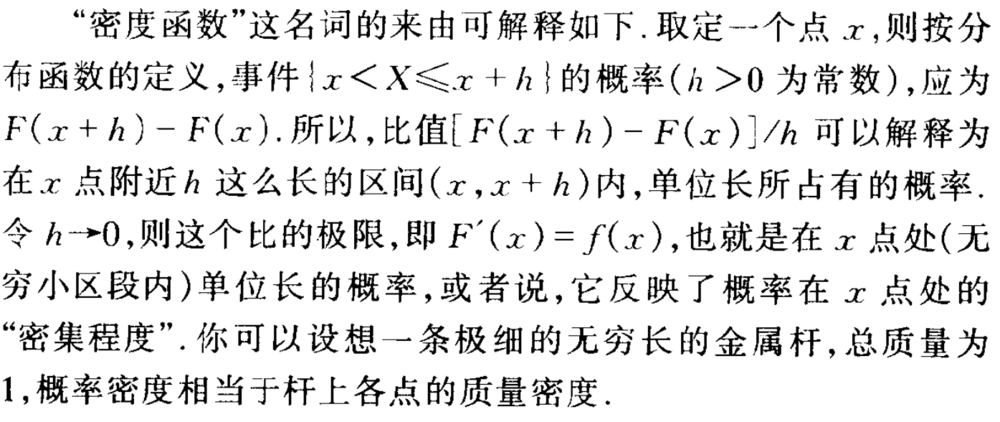如果这么解析你还是不太懂的话，看看下面的这个公式：

概率密度函数用数学公式表示就是一个定积分的函数，定积分在数学中是用来求面积的，而在这里，你就把概率表示为面积即可！

左边是F(x)连续型随机变量分布函数画出的图形，右边是f(x)连续型随机变量的概率密度函数画出的图像，它们之间的关系就是，概率密度函数是分布函数的导函数。

两张图一对比，你就会发现，如果用右图中的面积来表示概率，利用图形就能很清楚的看出，哪些取值的概率更大！所以，我们在表示连续型随机变量的概率时，用f(x)概率密度函数来表示，是非常好的！

但是，可能读者会有这样的问题：

Q：概率密度函数在某一点的值有什么意义？

A：比较容易理解的意义，某点的 概率密度函数 即为 概率在该点的变化率(或导数)。很容易误以为 该点概率密度值 为 概率值.

比如: 距离(概率)和速度(概率密度)的关系.

某一点的速度, 不能以为是某一点的距离
没意义,因为距离是从XX到XX的概念
所以, 概率也需要有个区间.
这个区间可以是x的邻域（可以无限趋近于0）。对x邻域内的f（x）进行积分，可以求得这个邻域的面积，就代表了这个邻域所代表这个事件发生的概率。

展开全文• 大学的时候，我的《概率论数理...今天我就讲讲应该如何理解概率分布函数概率密度函数的问题。是不是乍一看特别像，容易迷糊。如果你感到迷糊，恭喜你找到我当年的感觉了。 先从离散型随机变量连续性随机变量说起
• 连续型随机变量也有“概率函数”“概率分布函数”，只是连续型随机变量的“概率函数”叫“概率密度函数”。 概率密度函数用数学公式表示就是一个定积分的函数（如上），定积分在数学中是用来求面积的，而在这里可...机器学习
• 离散随机变量：随机变量的值可以都列举出来，则该随机变量称为离散型，例如，投掷骰子事件，该事件只出现两种情况：正面反面，可以使用整数01表示，0表示反面，1表示证明，则可以用离散随机变量来表示投掷骰子...
• 我用dfittool(x)拟合后的图像，发现服从下面的分布，请问如何对其进行拟合，求分布函数呀？ 捕获.PNG (22.65 KB, 下载次数: 8) 2016-8-30 16:00 上传 捕1获.PNG (22.32 KB, 下载次数: 8) 2016-8-30 16:03 上传
• http://www.360doc.com/content/17/0306/13/32342759_634411464.shtml什么是正态分布正态概率...某种产品的尺寸质量；降雨量；学习成绩，特别是，在统计推断时，当样本的数量足够大时，许多统计数据都服从正态分布...
• t分布的定义和概率密度函数

千次阅读 2021-07-02 14:05:31
定义: 设 X1∼N(0,1),X2∼χ2(n)X_{1} \sim N(0,1), X_{2} \sim \chi^{2}(n)X1​∼N(0,1),X2​∼χ2(n) , 且 X1...所服从的分布为自由度为 nnn 的 $t$ 分布.记为 t∼t(n){t} \sim{t}({n})t∼t(n) 概率密度函数： p(y)=
• 定义: 设 X1∼χ2(m),X2∼χ2(n)X_{1} \sim \chi^{2}(m), X_{2} \sim \chi^{2}(n)X1​∼χ2(m),X2​∼χ2(n), X1X_{1}X1...服从自由度为 mmm 及 nnn 的 FFF 分布， mmm 称为第一自由度, n\boldsymbol{n}n 称为第二自由
• 概率分布和概率函数只对离散型变量有意义，那如何描述连续型变量呢？ 答案就是“概率分布函数F(x)”和“概率密度函数f(x)”，当然这两者也是可以描述离散型变量的。 概率分布函数F(x)：给出取值小于某个值的概率，是...
• 概率密度分布函数上分位点的数值计算.doc 1 大连民族学院 数 学 实 验 报 告 课程： 数理统计 实验题目: 概率密度、分布函数上分位点的数值计算 系别： 理学院 专业： 信息与计算科学 姓名： 历红影 班级： 信息...
• 标准正态分布密度函数公式

千次阅读 2021-02-01 03:17:02
展开全部标准正态分布密度函数公式：正态曲线呈钟型62616964757a686964616fe58685e5aeb931333366306532，两头低，中间高，左右对称因其曲线呈钟形，因此人们又经常称之为钟形曲线。若随机变量X服从一个数学期望为μ...
• 概率密度分布函数上分位点的数值计算 1 大连民族学院 数 学 实 验 报 告 课程： 数理统计 实验题目: 概率密度、分布函数上分位点的数值计算 系别： 理学院 专业： 信息与计算科学 姓名： 历红影 班级： 信息102...
• 离散型随机变量及分布分布名称 记法 分布律 均值E(X)E(X)E(X) 方差D(X)D(X)D(X) 伯努利分布 X∼B(p)X\thicksim B(p)X∼B(p) P(X=k)=pk(1−p)1−k,k=0,1P(X=k)=p^k(1-p)^{1-k},\quad k=0,1P(X=k)=pk(1−p)...
• 该模块程序为：function y = crnd(pdffun, pdfdef, m, n)%生成任意一元连续分布随机数% y = crnd(pdffun, pdfdef, m, n)，产生指定一元连续分布的随机数，m行n列。pdffun为密度% 函数表达式，pdfdef为密度函数定义域...
• 自变量是随机变量，它可以取很多值，每个值...概率函数概率分布、概率密度是一样的，只是说用函数表达式写出来而已。 分布函数前面三个有点不太一样，分布函数某一点的值是该点前面所有概率的累加。 分布函...
• F分布的概率密度函数如下图所示：其中：μ为分子自由度，ν为分母自由度Γ为伽马函数的的符号由于Excel没有求F分布的概率密度函数可用，但是F分布中涉及到GAMMALN()函数，而excel是提供GAMMALN()函数的，所以我们...
• 上一篇文章写到如何利用ArcGIS...这篇文章来点不一样的，也是我感觉挺有意思的，就是利用ArcGIS绘制一种与众不同的人口分布密度图；在学习本文内容之前呢，小伙伴们首先得会利用ArcGIS创建渔网，需要上一篇基础上 ...arcgis 爬虫
• lognormal分布、瑞利分布、nakagami分布 概率密度函数仿真 x=0:0.1:15; y1=lognpdf(x,0.5,1); yy2=raylpdf(x,1); mu=1;omega=2; f=@(x)((2*mu.^mu.*x.^(2*mu-1).*exp(-mu*x.^2./omega)./gamma(mu)./omega^mu).*(x&...matlab
• 概率密度分布和逆概率分布函数值的计算MATLAB统计工具箱中有这样一系列函数，函数名以pdf三个字符结尾的函数用来计算常见连续分布的密度函数值或离散分布的概率函数值，函数名以cdf三个字符结尾的函数用来计算常见...
• 高斯分布的概率密度函数 f(x)=12πσexp((x−μ)22σ2). f(x)=\frac{1}{\sqrt{2\pi }\sigma }exp(\frac{(x-\mu )^{2}}{2\sigma ^{2}}). f(x)=2π​σ1​exp(2σ2(x−μ)2​). numpy中 numpy.random.normal(loc=0.0, ...python
• 概率分布F(x)和概率密度f(x)

千次阅读 2021-03-17 13:47:02
概率分布函数概率密度函数1、概率密度函数2、概率质量函数3、累积分布函数4、常用概率密度函数    从数学上看，分布函数F(x)=P(X<x)，表示随机变量X的值小于x的概率。这个意义很容易理解。概率密度f(x)是F(x)...
• 首先，把二维正态分布密度函数的公式贴这里 这只图好大啊~~但是上面的那个是多维正态分布密度函数的通式，那个n阶是对称正定方阵叫做协方差矩阵，其中的x,pi,u都是向量形式。虽然这个式子很酷，但是用在matlab里...
• MATLAB如何使用pdf函数计算指定分布的概率密度函数【语法说明】Y=pdf(‘name’,X,A)Y=pdf(‘name’,X,A,B)Y=pdf(‘name’,X,A,B,C)字符串name指定概率分布的种类，函数计算自变量X在指定概率分布下的概率值，A、B、C...
• MATLAB如何使用wblpdf函数计算韦伯分布的概率密度【语法说明】Y=wblpdf(X,A,B)：计算X中的元素在参数A、B指定的韦伯分布下的概率密度函数值。Y 是与 X、A、B 同型的数组，如果输入参数中有一个为标量，则将其扩展为...matlab韦伯分布
• 正太分布和概率密度函数，期望值，方差 正态分布(Normal distribution)，又名高斯分布（Gaussian distribution）是一个非常常见的连续概率分布。正态分布在统计学上十分重要，经常用在自然和社会科学来代表一个不明...统计学
• 使用Excel绘制t分布概率密度函数关于t分布应用广泛，主要用于假设检验。关于使用Excel画出t分布的概率密度函数图表的问题，试答如下：使用excel绘制t分布的概率密度函数，需要两列：1)自变量X，2)计算自变量X对应的t......